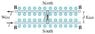# Sliding Rod In A Magnetic Field (w/ Static Friction)

• SMA83

## Homework Statement

Suppose the rod in the figure has mass m= 0.44 kg and length 26 cm and the current through it is I= 40 A.

Part A: If the coefficient of static friction is mus= 0.45, determine the minimum magnetic field B (not necessarily vertical) that will just cause the rod to slide. Give the magnitude of B.

Part B: Give the direction of B relative to the vertical (outwards towards us).

## Homework Equations

F=I*L*B*sin(theta)
Ffriction=Fn*mus
Fn=m*g

## The Attempt at a Solution

This problem seemed straightforward until they mentioned that the magnetic field isn't necessarily vertical. I am able to plug in the numbers and calculate a solution with a vertical megnetic field, but when I have two unknowns (theta and B), I am not sure how to find the angle that would require the smallest value for B.

Note: There is already a thread based on a problem similar to this one here: https://www.physicsforums.com/showthread.php?t=303237. I didn't find the explanation sufficient to get me through the problem, however...I guess I'm a little slower than the guy in that thread. Thanks in advance for any help.

Without the diagram it is hard to be specific, but the idea is to set the magnetic force equal to the friction force and then fill in the detailed force formulas so you can solve for B. The B field will have a component (something like B*sin(A)) in the magnetic force formula and the other component (perhaps B*cos(A) ) will tend to lift the rod, reducing the normal force in the friction formula.

I expect you will end up with B = an expression with some constants and the angle. You could graph the expression as a function of the angle to find what angle gives the minimum value of the magnetic field.

Oops, I forgot to upload the image. I'll include it as an attachment here.

So, following what you said, I found:

FN=mg-ILBsin(theta)

which means:

Ffriction=mus(mg-ILBsin(theta))

therefore:

ILBcos(theta)=mus(mg-ILBsin(theta))

Does it look like I'm on the right track so far?

#### Attachments

•GIANCOLI_ch27_p59_60.jpg
7.2 KB · Views: 1,055
Looks good!

Well, I think I must have done it right because the answer is coming out correctly now. The final equation I put into my calculator look like:

(mus*m*g)/(ILcos(theta)+musILsin(theta))

Of course, I filled in the known variables, which was everything but theta, and used theta as the x-value in my graph. Then I used the minimum value function on my calculator to find where the minimum was, and when rounded to 2 sig figs, masteringphysics seemed to like it. Thanks a lot for your help...I was pretty lost on this one.